# matplotlib.colors.LinearSegmentedColormap#

class matplotlib.colors.LinearSegmentedColormap(name, segmentdata, N=256, gamma=1.0)[source]#

Bases: Colormap

Colormap objects based on lookup tables using linear segments.

The lookup table is generated using linear interpolation for each primary color, with the 0-1 domain divided into any number of segments.

Create colormap from linear mapping segments

segmentdata argument is a dictionary with a red, green and blue entries. Each entry should be a list of x, y0, y1 tuples, forming rows in a table. Entries for alpha are optional.

Example: suppose you want red to increase from 0 to 1 over the bottom half, green to do the same over the middle half, and blue over the top half. Then you would use:

cdict = {'red':   [(0.0,  0.0, 0.0),
(0.5,  1.0, 1.0),
(1.0,  1.0, 1.0)],

'green': [(0.0,  0.0, 0.0),
(0.25, 0.0, 0.0),
(0.75, 1.0, 1.0),
(1.0,  1.0, 1.0)],

'blue':  [(0.0,  0.0, 0.0),
(0.5,  0.0, 0.0),
(1.0,  1.0, 1.0)]}


Each row in the table for a given color is a sequence of x, y0, y1 tuples. In each sequence, x must increase monotonically from 0 to 1. For any input value z falling between x[i] and x[i+1], the output value of a given color will be linearly interpolated between y1[i] and y0[i+1]:

row i:   x  y0  y1
/
/
row i+1: x  y0  y1


Hence y0 in the first row and y1 in the last row are never used.

LinearSegmentedColormap.from_list

Static method; factory function for generating a smoothly-varying LinearSegmentedColormap.

static from_list(name, colors, N=256, gamma=1.0)[source]#

Create a LinearSegmentedColormap from a list of colors.

Parameters:
namestr

The name of the colormap.

colorsarray-like of colors or array-like of (value, color)

If only colors are given, they are equidistantly mapped from the range $$[0, 1]$$; i.e. 0 maps to colors and 1 maps to colors[-1]. If (value, color) pairs are given, the mapping is from value to color. This can be used to divide the range unevenly.

Nint

The number of RGB quantization levels.

gammafloat
resampled(lutsize)[source]#

Return a new colormap with lutsize entries.

reversed(name=None)[source]#

Return a reversed instance of the Colormap.

Parameters:
namestr, optional

The name for the reversed colormap. If None, the name is set to self.name + "_r".

Returns:
LinearSegmentedColormap

The reversed colormap.

set_gamma(gamma)[source]#

Set a new gamma value and regenerate colormap.

## Examples using matplotlib.colors.LinearSegmentedColormap#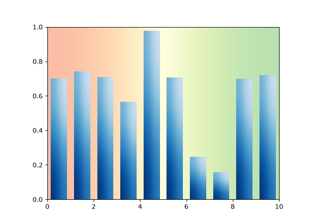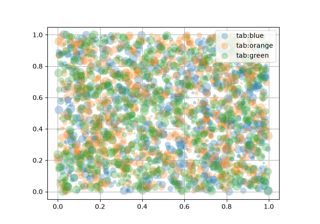Scatter plots with a legend

Scatter plots with a legend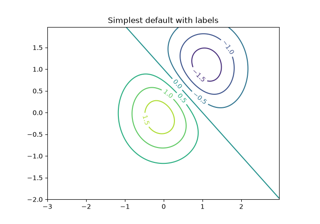Contour Demo

Contour Demo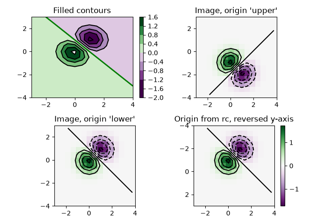Contour Image

Contour Image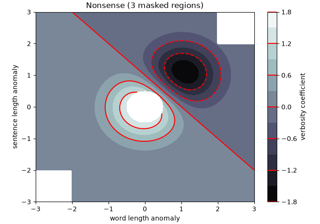Contourf demo

Contourf demo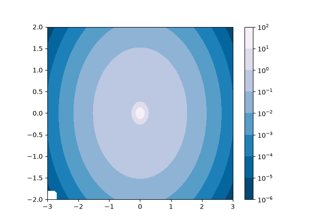Contourf and log color scale

Contourf and log color scale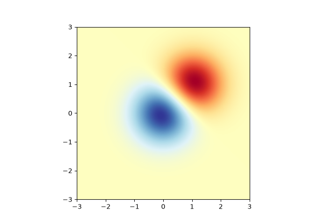Image demo

Image demo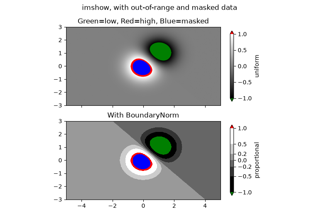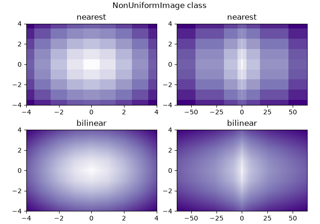Image nonuniform

Image nonuniform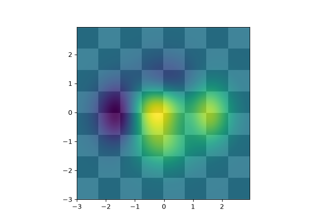Layer Images

Layer Images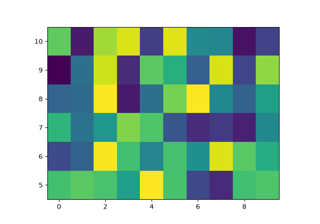pcolormesh

pcolormesh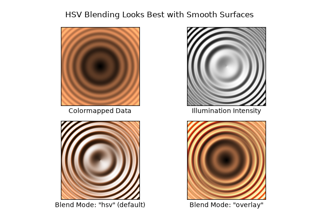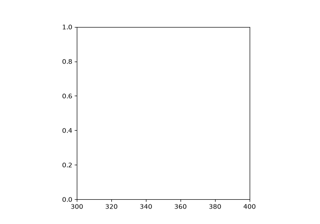Axes box aspect

Axes box aspect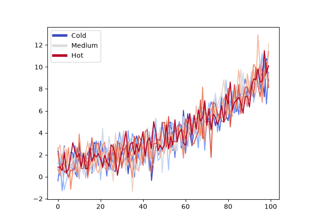Composing Custom Legends

Composing Custom Legends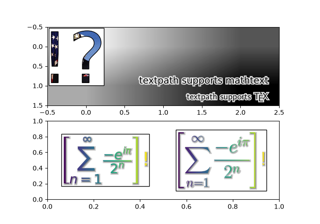Using a text as a Path

Using a text as a Path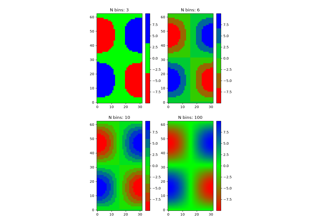Creating a colormap from a list of colors

Creating a colormap from a list of colors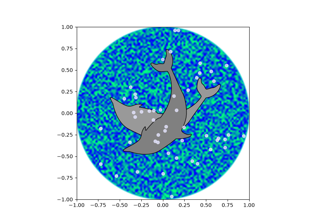Dolphins

Dolphins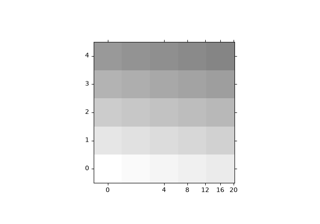Demo CurveLinear Grid2

Demo CurveLinear Grid2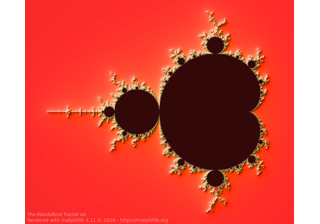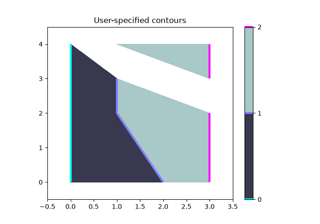Manual Contour

Manual Contour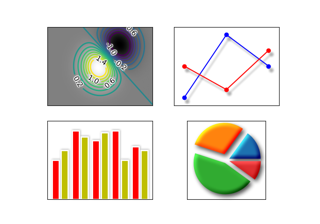AGG filter

AGG filter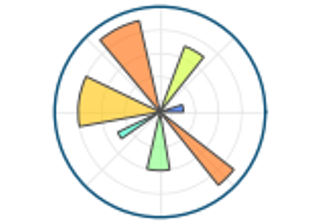Matplotlib logo

Matplotlib logo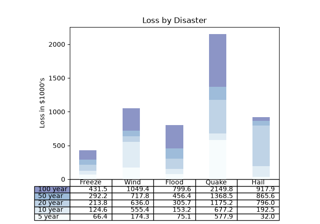Table Demo

Table Demo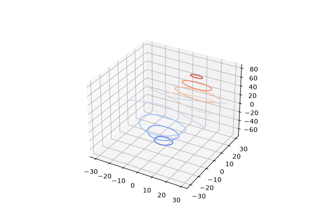Plot contour (level) curves in 3D

Plot contour (level) curves in 3D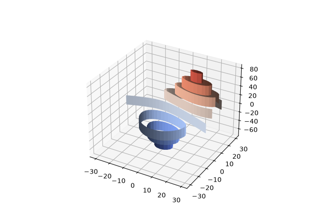Plot contour (level) curves in 3D using the extend3d option

Plot contour (level) curves in 3D using the extend3d option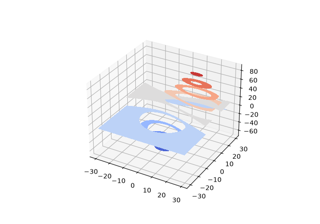Filled contours

Filled contours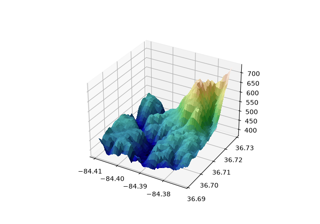Custom hillshading in a 3D surface plot

Custom hillshading in a 3D surface plot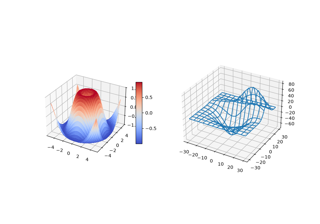3D plots as subplots

3D plots as subplots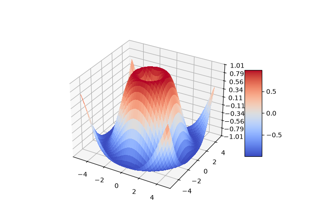3D surface (colormap)

3D surface (colormap)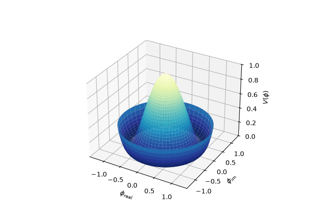3D surface with polar coordinates

3D surface with polar coordinates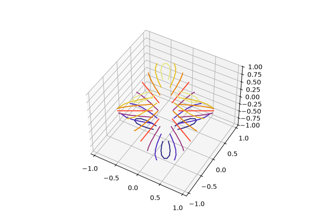Triangular 3D contour plot

Triangular 3D contour plot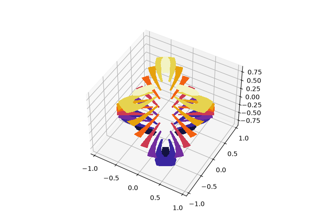Triangular 3D filled contour plot

Triangular 3D filled contour plot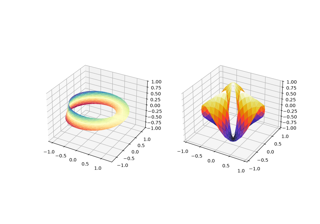More triangular 3D surfaces

More triangular 3D surfaces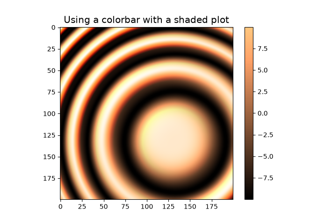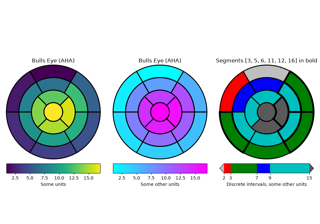Left ventricle bullseye

Left ventricle bullseye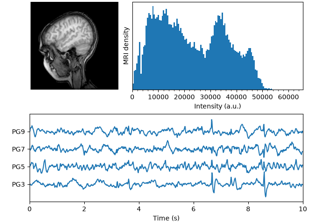MRI with EEG

MRI with EEG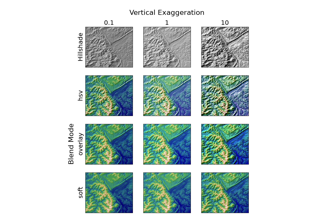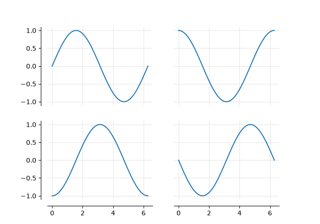Dropped spines

Dropped spinesColorbar Tick Labelling

Colorbar Tick Labelling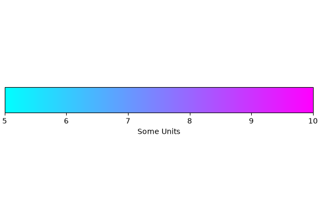Customized Colorbars Tutorial

Customized Colorbars Tutorial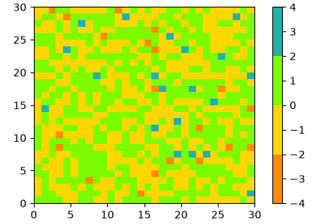Creating Colormaps in Matplotlib

Creating Colormaps in Matplotlib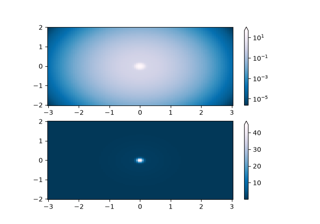Colormap Normalization

Colormap Normalization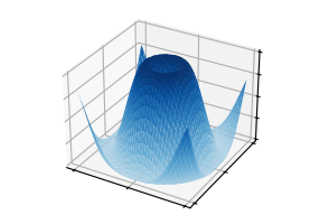3D surface

3D surface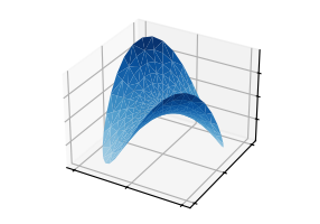Triangular 3D surfaces

Triangular 3D surfaces